# Chapter 11 Liquids solids and intermolecular forces Intermolecular

• Slides: 45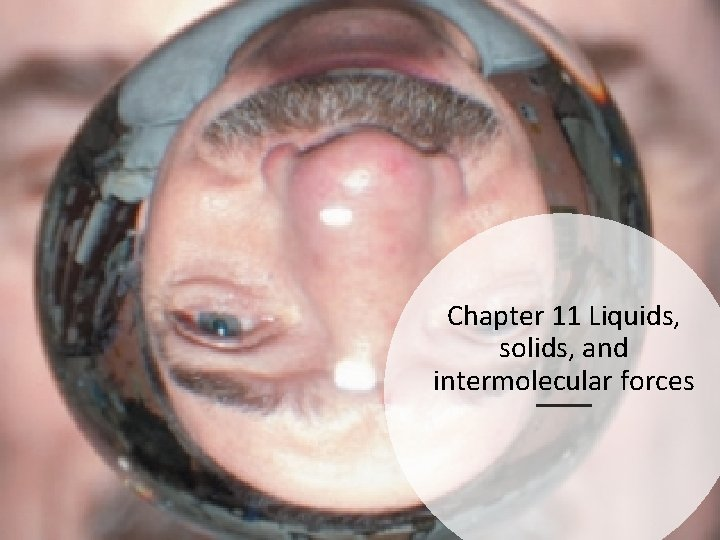Chapter 11 Liquids, solids, and intermolecular forces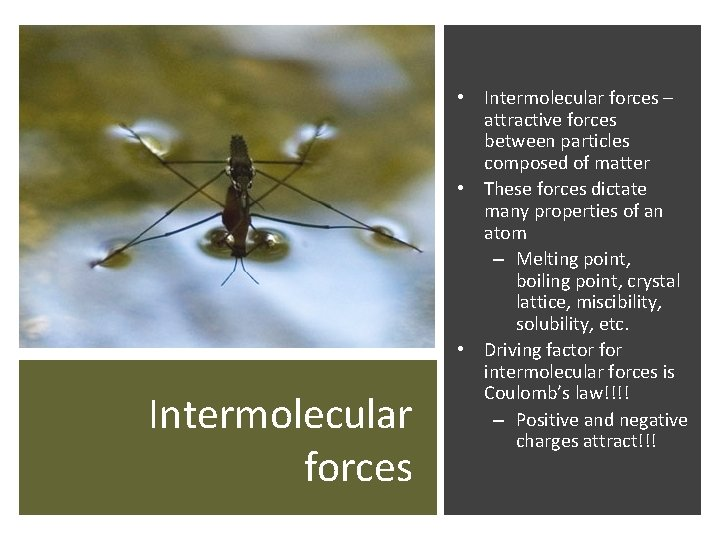Intermolecular forces • Intermolecular forces – attractive forces between particles composed of matter • These forces dictate many properties of an atom – Melting point, boiling point, crystal lattice, miscibility, solubility, etc. • Driving factor for intermolecular forces is Coulomb’s law!!!! – Positive and negative charges attract!!!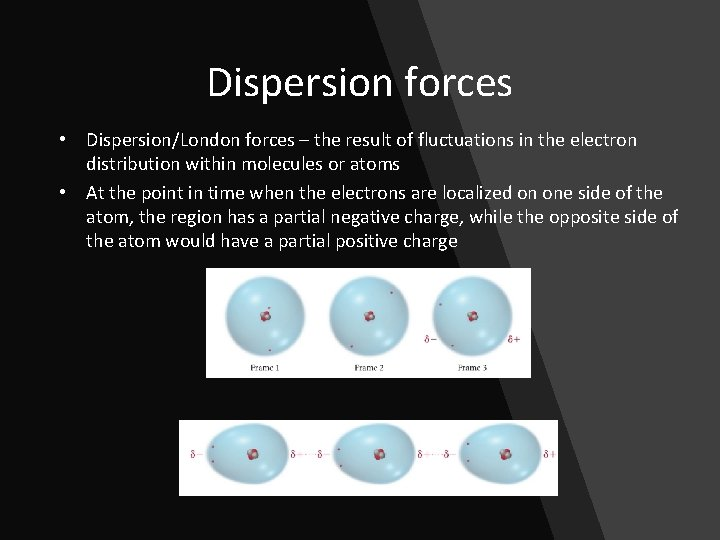Dispersion forces • Dispersion/London forces – the result of fluctuations in the electron distribution within molecules or atoms • At the point in time when the electrons are localized on one side of the atom, the region has a partial negative charge, while the opposite side of the atom would have a partial positive chargeDispersion force: Atom size • Larger atoms will have greater dispersion forces – More electrons, more space, more volume in which electrons can polarize the atom • Greater dispersion force results in higher melting points and boiling points since it take more energy (higher temperature) to separate the attracted particles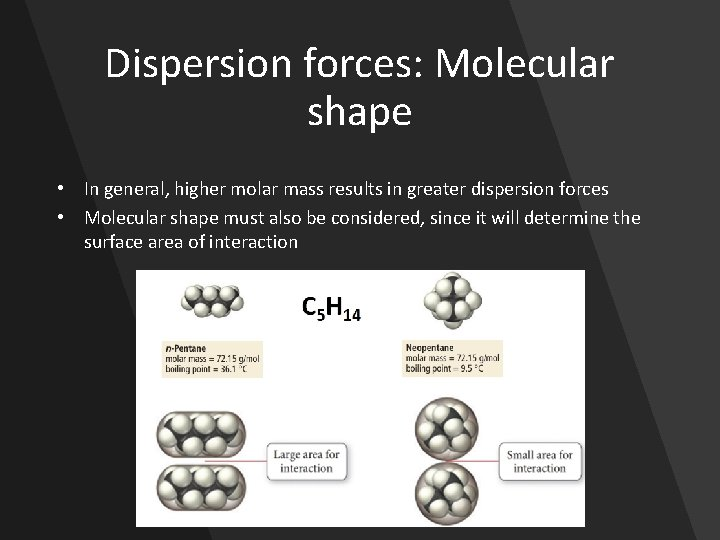Dispersion forces: Molecular shape • In general, higher molar mass results in greater dispersion forces • Molecular shape must also be considered, since it will determine the surface area of interaction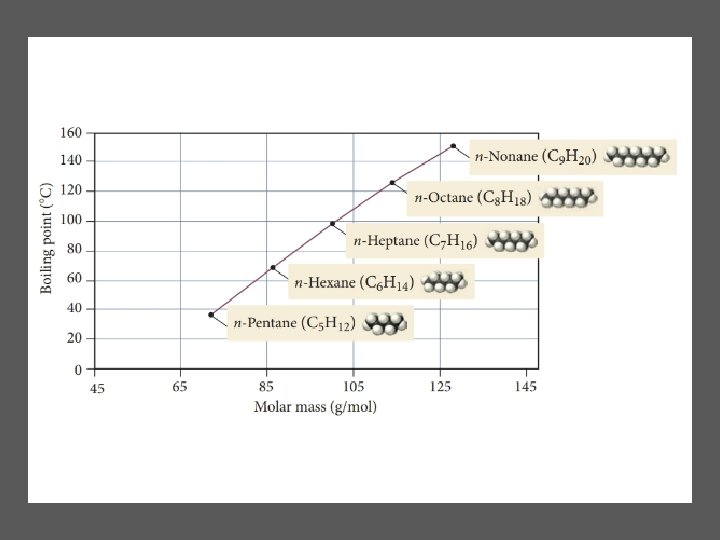Dipole-dipole force • Dipole-dipole force – attractive force between polar molecules – If a molecule has a net dipole it is polar and the positive region of one molecule can attract to the negative region in another molecule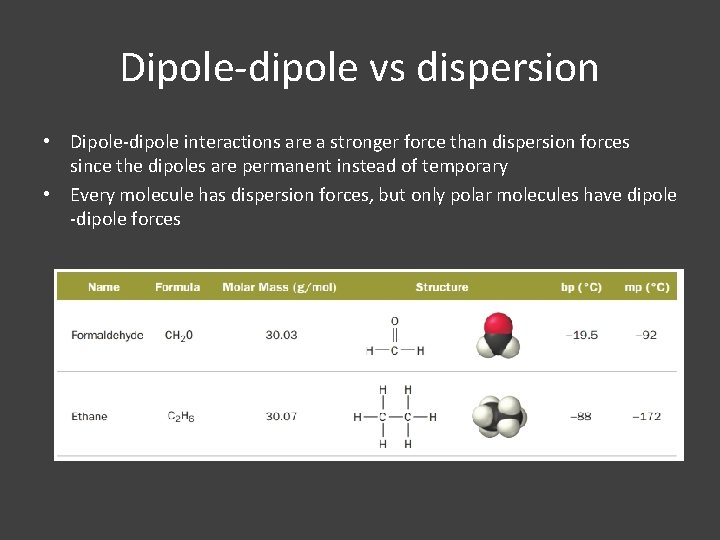Dipole-dipole vs dispersion • Dipole-dipole interactions are a stronger force than dispersion forces since the dipoles are permanent instead of temporary • Every molecule has dispersion forces, but only polar molecules have dipole -dipole forcesDipole-dipole force: Dipole moment • The greater the magnitude of the positive and negative charge in the dipole, the greater the force • Increasing dipole moment = stronger dipole-dipole force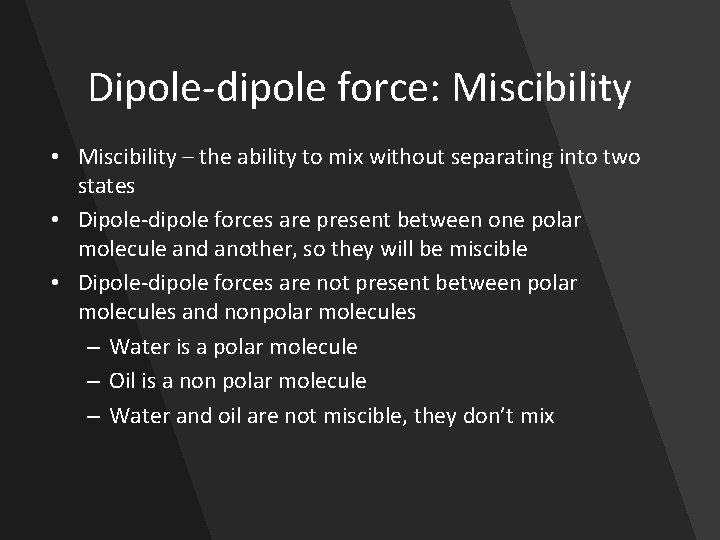Dipole-dipole force: Miscibility • Miscibility – the ability to mix without separating into two states • Dipole-dipole forces are present between one polar molecule and another, so they will be miscible • Dipole-dipole forces are not present between polar molecules and nonpolar molecules – Water is a polar molecule – Oil is a non polar molecule – Water and oil are not miscible, they don’t mix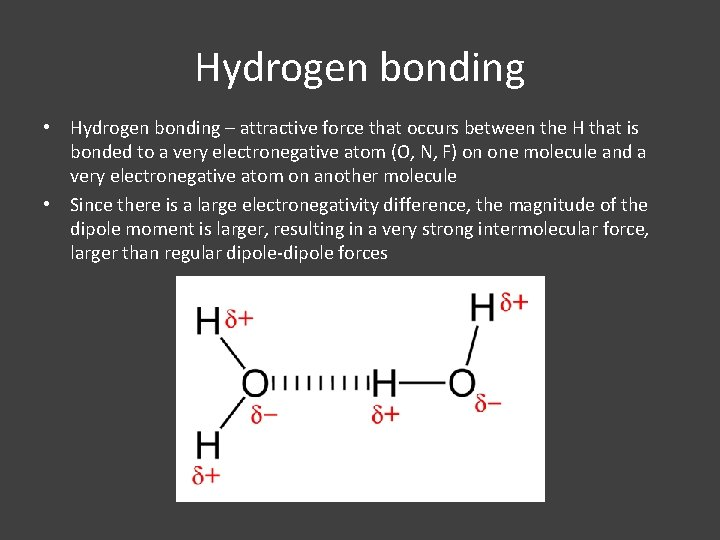Hydrogen bonding • Hydrogen bonding – attractive force that occurs between the H that is bonded to a very electronegative atom (O, N, F) on one molecule and a very electronegative atom on another molecule • Since there is a large electronegativity difference, the magnitude of the dipole moment is larger, resulting in a very strong intermolecular force, larger than regular dipole-dipole forces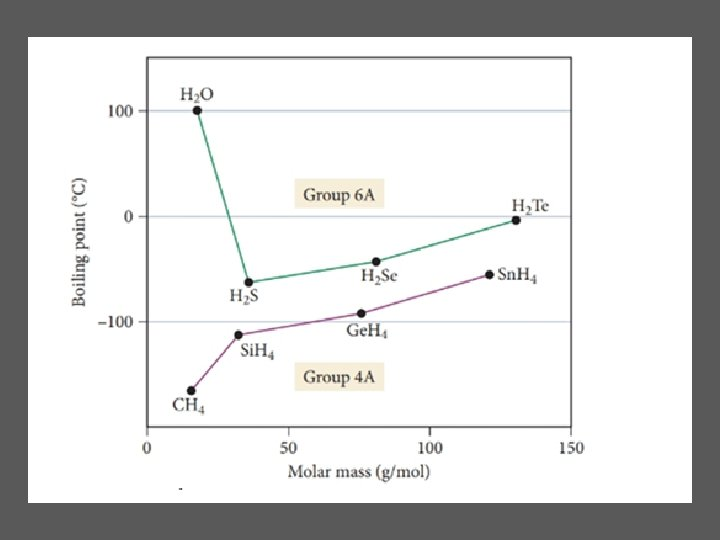Ion-dipole force • • Ion-dipole force – attractive force between ions and a polar compound Ionic compound composed of cation and anion – Cation is attracted to negative end of dipole – Anion is attracted to positive end of dipole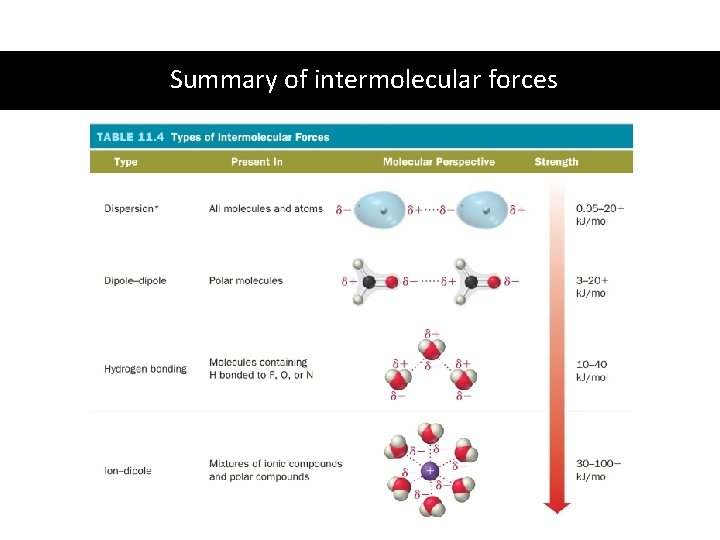Summary of intermolecular forcesPractice Identify the intermolecular forces at play in a sample of the following molecules (assume solid or liquid phase) • Cl 2 • NH 3 • KI Identify the major interaction expected between the following molecules • Water and ammonia (NH 3) • Formaldehyde (CH 2 O) and methane (CH 4)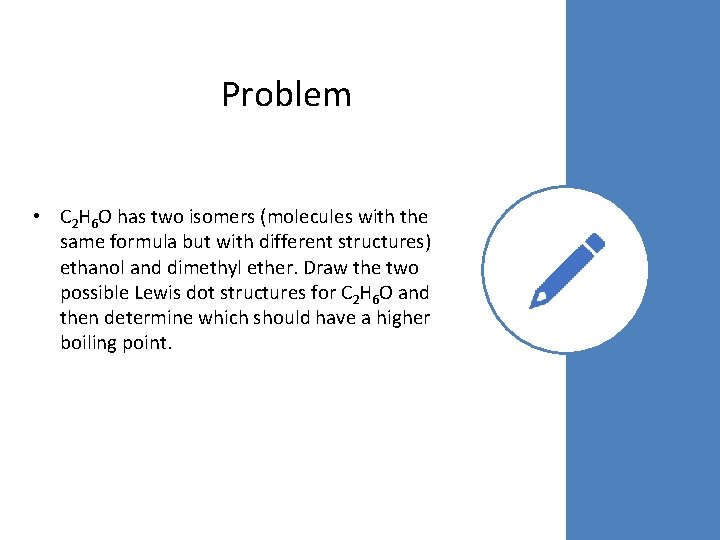Problem • C 2 H 6 O has two isomers (molecules with the same formula but with different structures) ethanol and dimethyl ether. Draw the two possible Lewis dot structures for C 2 H 6 O and then determine which should have a higher boiling point.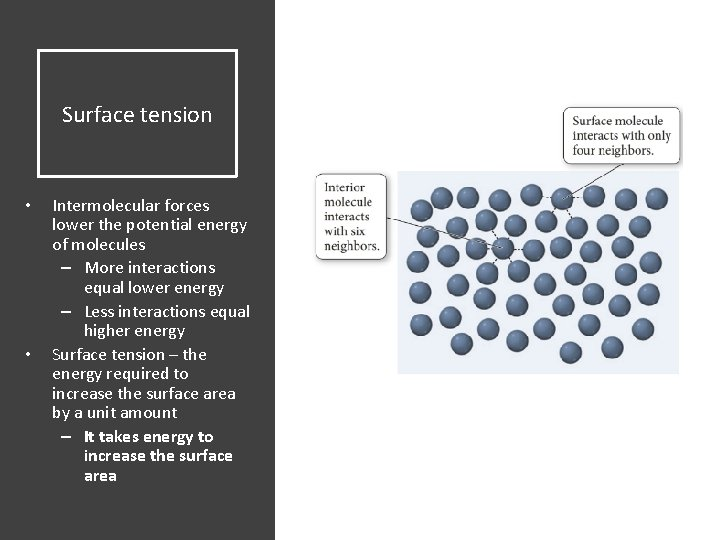Surface tension • • Intermolecular forces lower the potential energy of molecules – More interactions equal lower energy – Less interactions equal higher energy Surface tension – the energy required to increase the surface area by a unit amount – It takes energy to increase the surface area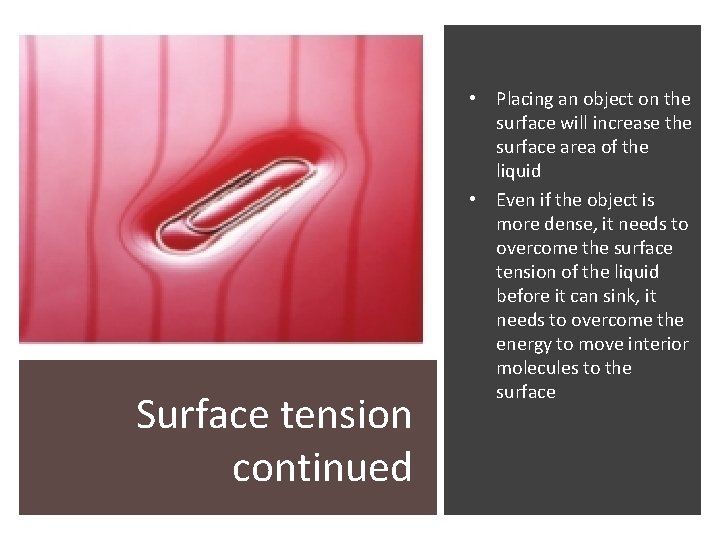Surface tension continued • Placing an object on the surface will increase the surface area of the liquid • Even if the object is more dense, it needs to overcome the surface tension of the liquid before it can sink, it needs to overcome the energy to move interior molecules to the surfaceViscosity • • Intermolecular forces attract molecules to each other, making them less likely to flow Viscosity – the resistance of a liquid to flow – Lower viscosity, faster flow • Water – Higher viscosity, slower flow • Maple syrup All factors that enhance intermolecular forces (force type, shape and size) will affect viscosity Temperature also affects viscosity – Higher temperature, lower viscosity – Lower temperature, higher viscosityViscosity trendsCapillary action • Capillary action – the ability of a liquid to flow against gravity up a narrow tube • Adhesive force – the attraction of the molecules with the surface of the tube • Cohesive force – the attraction of the molecules in the liquid to each other • Adhesive forces pull the liquid up a tube until gravity balances out the capillary actionTube width and meniscus shape • • • Liquid will travel higher up more narrower tubes – Narrower tube increases adhesive forces per volume of liquid Stronger adhesive forces cause liquid to creep up the sides to produce a concave meniscus shape If cohesive forces are stronger then the meniscus will have a convex shape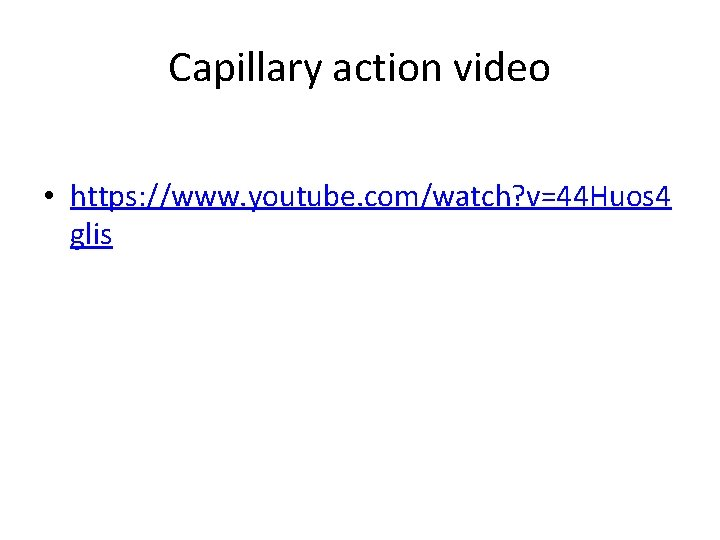Capillary action video • https: //www. youtube. com/watch? v=44 Huos 4 glis• Vaporization – the transition from liquid to gas • The thermal energy of molecules in a sample is represented by a distribution curve that has a dependence on temperature • Molecules on the higher end of the distribution curve can have enough energy to transition into the gas phase VaporizationHeat of vaporization ΔHvap • • It requires energy to go from the liquid to the gas phase (to vaporize), this process is endothermic Heat of vaporization (ΔHvap) – the amount of heat required to vaporize one mole of a liquid to a gas • At 100° C (boiling point of water) – ΔHvap = +40. 7 k. J/mol H 2 O(l) H 2 O(g)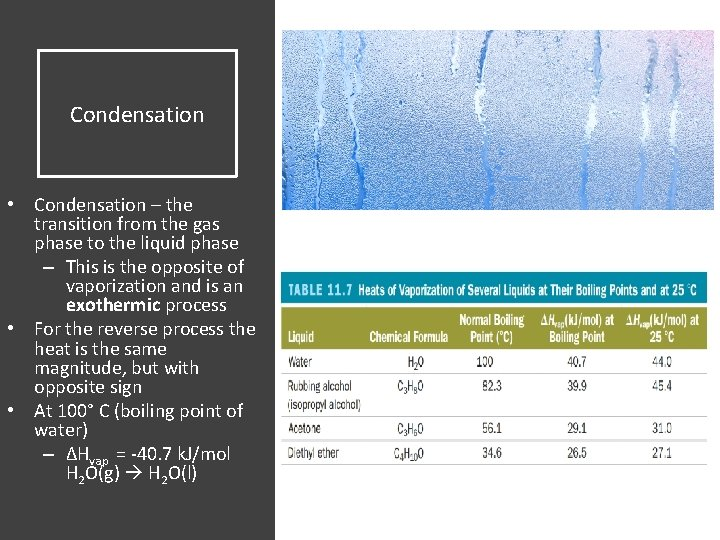Condensation • Condensation – the transition from the gas phase to the liquid phase – This is the opposite of vaporization and is an exothermic process • For the reverse process the heat is the same magnitude, but with opposite sign • At 100° C (boiling point of water) – ΔHvap = -40. 7 k. J/mol H 2 O(g) H 2 O(l)How much heat does it take to boil 1 mol of water at its boiling point? Practice How much heat does it take to boil 45. 8 g of water at its boiling point? Calculate the mass of water (in grams) that can be vaporized at its boiling point with 155 k. J of heat.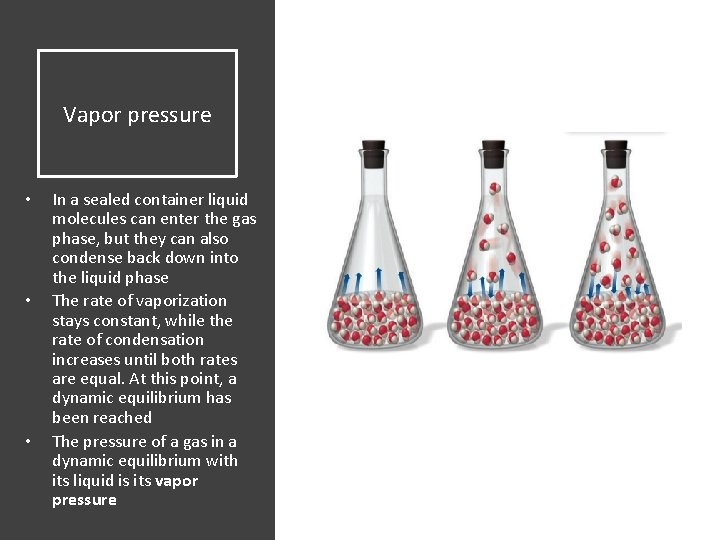Vapor pressure • • • In a sealed container liquid molecules can enter the gas phase, but they can also condense back down into the liquid phase The rate of vaporization stays constant, while the rate of condensation increases until both rates are equal. At this point, a dynamic equilibrium has been reached The pressure of a gas in a dynamic equilibrium with its liquid is its vapor pressure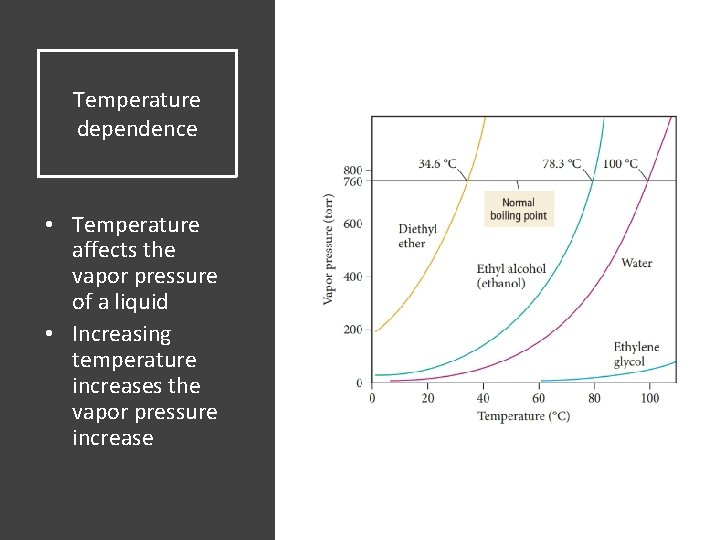Temperature dependence • Temperature affects the vapor pressure of a liquid • Increasing temperature increases the vapor pressure increase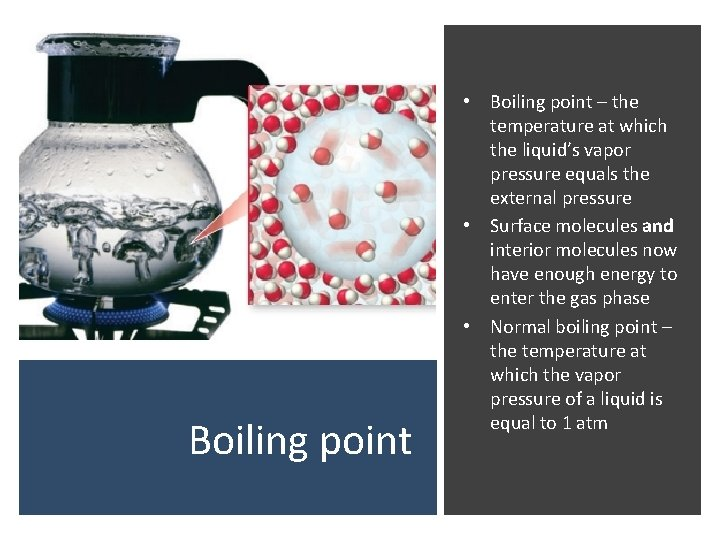Boiling point • Boiling point – the temperature at which the liquid’s vapor pressure equals the external pressure • Surface molecules and interior molecules now have enough energy to enter the gas phase • Normal boiling point – the temperature at which the vapor pressure of a liquid is equal to 1 atmPressure dependence • Lower pressure will lower the boiling point • Lower pressure makes it easier for liquid molecules to enter the gas phase, lowering the temperature at which the liquid “boils”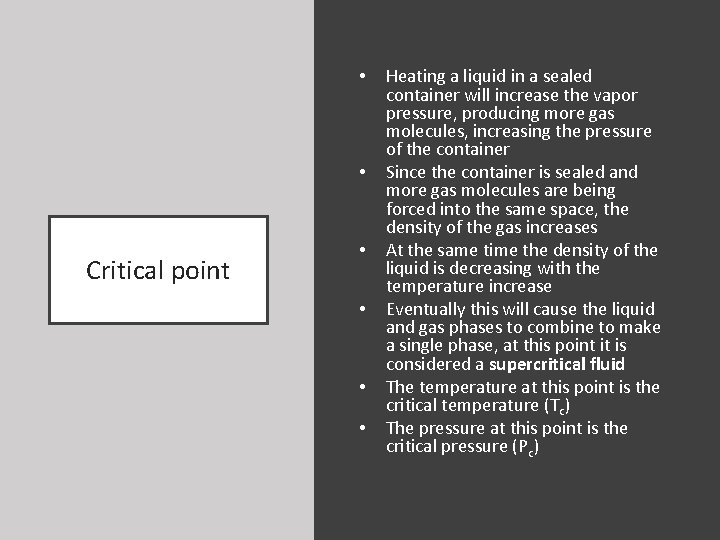• • Critical point • • Heating a liquid in a sealed container will increase the vapor pressure, producing more gas molecules, increasing the pressure of the container Since the container is sealed and more gas molecules are being forced into the same space, the density of the gas increases At the same time the density of the liquid is decreasing with the temperature increase Eventually this will cause the liquid and gas phases to combine to make a single phase, at this point it is considered a supercritical fluid The temperature at this point is the critical temperature (Tc) The pressure at this point is the critical pressure (Pc)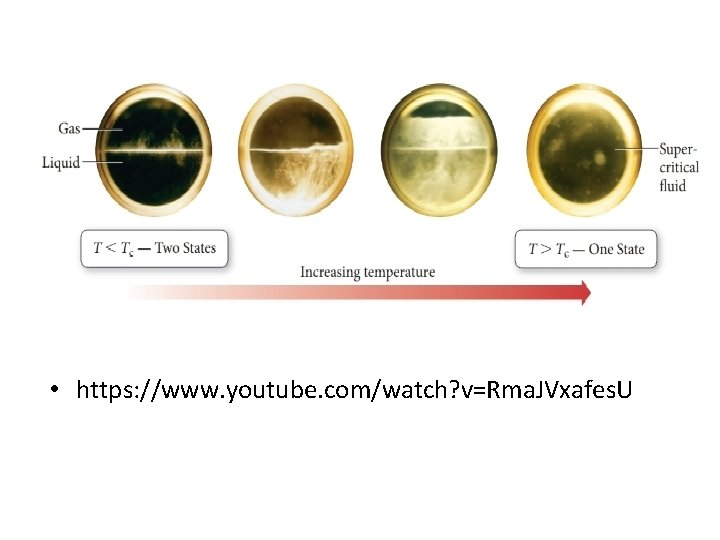• https: //www. youtube. com/watch? v=Rma. JVxafes. UHeating a liquid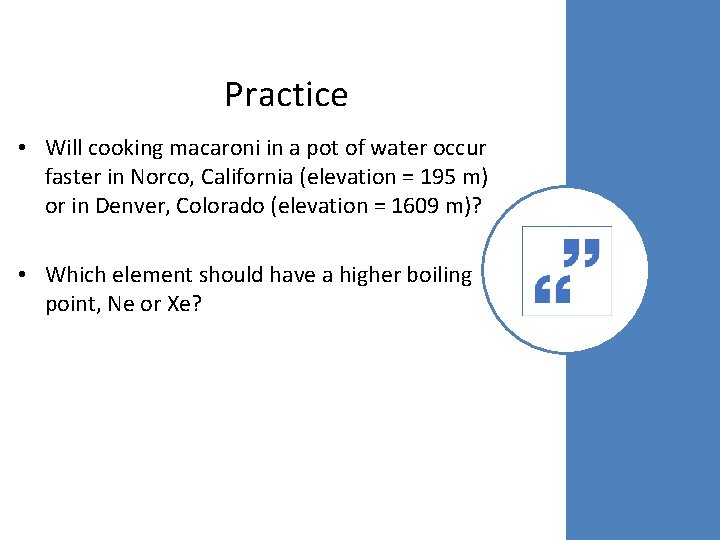Practice • Will cooking macaroni in a pot of water occur faster in Norco, California (elevation = 195 m) or in Denver, Colorado (elevation = 1609 m)? • Which element should have a higher boiling point, Ne or Xe?Solid phase changes • • • Sublimation – transition from the solid to gas phase Deposition – transition from gas to solid phase Melting/fusion – transition of a solid to liquid phase Freezing – the transition of a liquid to a solid Melting/freezing point – the temperature at which a liquid freezes, or a solid melts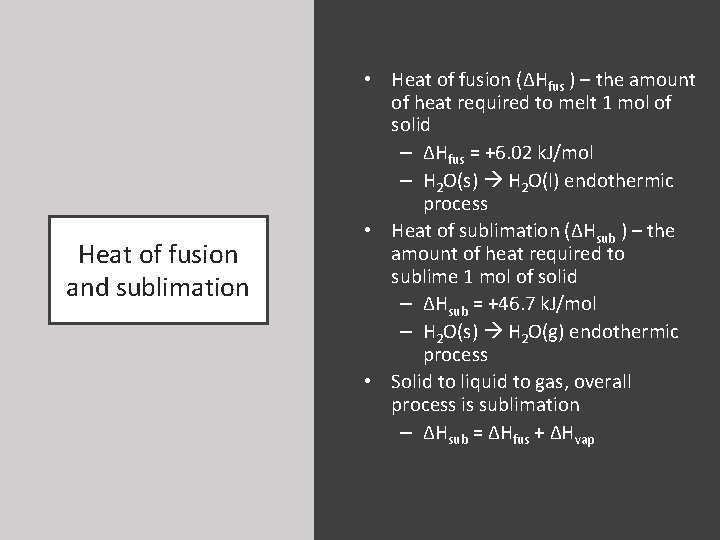Heat of fusion and sublimation • Heat of fusion (ΔHfus ) – the amount of heat required to melt 1 mol of solid – ΔHfus = +6. 02 k. J/mol – H 2 O(s) H 2 O(l) endothermic process • Heat of sublimation (ΔHsub ) – the amount of heat required to sublime 1 mol of solid – ΔHsub = +46. 7 k. J/mol – H 2 O(s) H 2 O(g) endothermic process • Solid to liquid to gas, overall process is sublimation – ΔHsub = ΔHfus + ΔHvap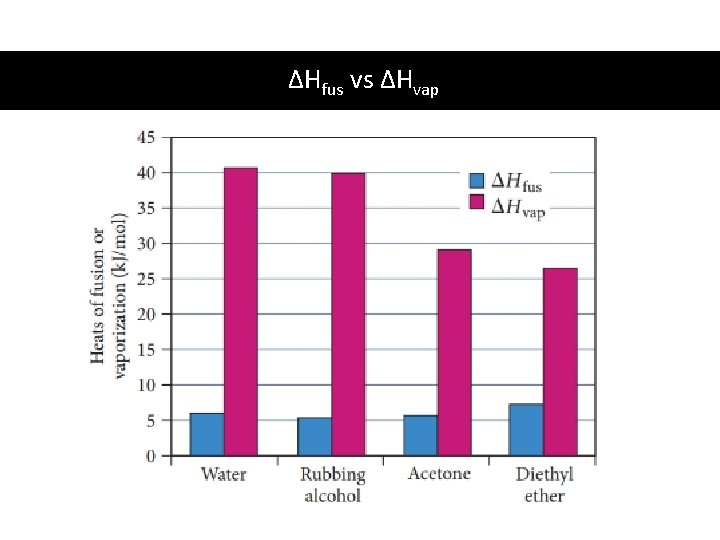ΔHfus vs ΔHvap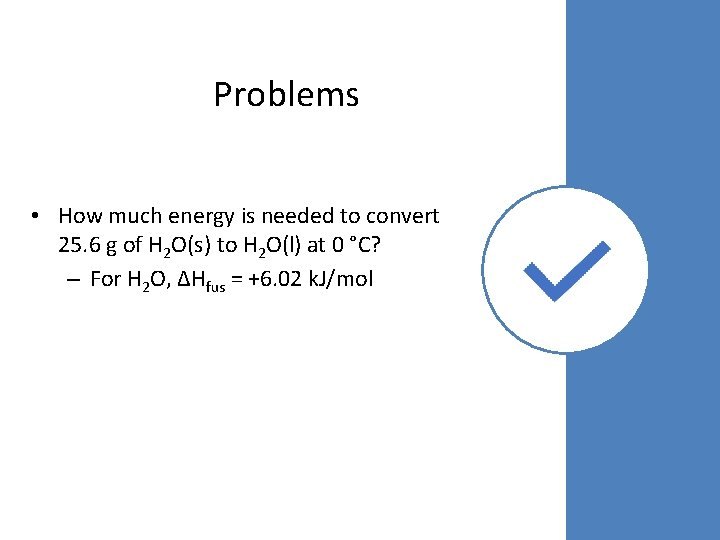Problems • How much energy is needed to convert 25. 6 g of H 2 O(s) to H 2 O(l) at 0 °C? – For H 2 O, ΔHfus = +6. 02 k. J/mol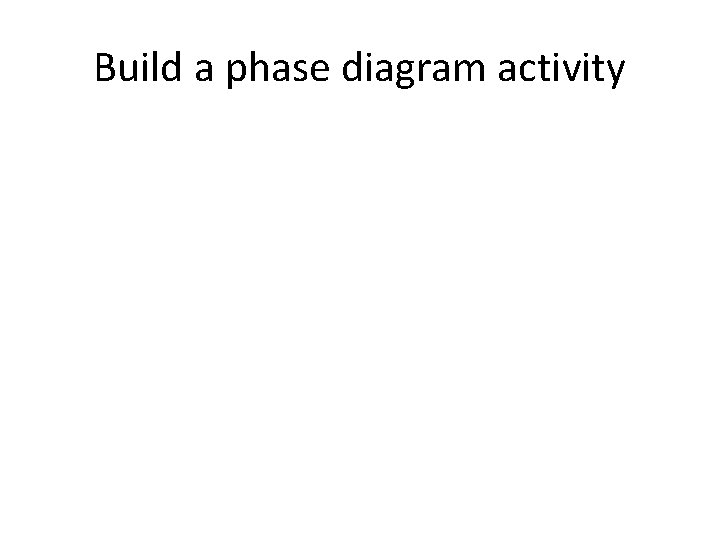Build a phase diagram activityPhase diagrams • • Temperature and pressure affect the phase of a substance Phase diagrams show at a given temperature and pressure what a phase will be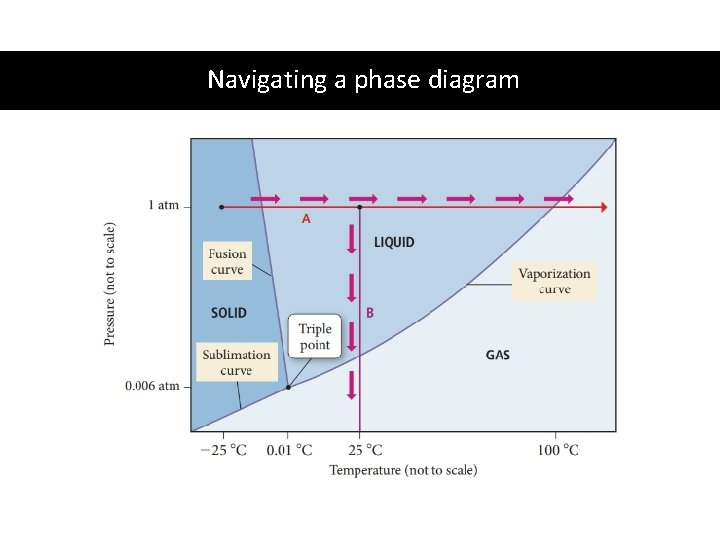Navigating a phase diagramPhase diagrams • Intermolecular forces for different substances change the properties of that substance (melting point, boiling point, critical point, triple point) • Phase diagrams are different for each substance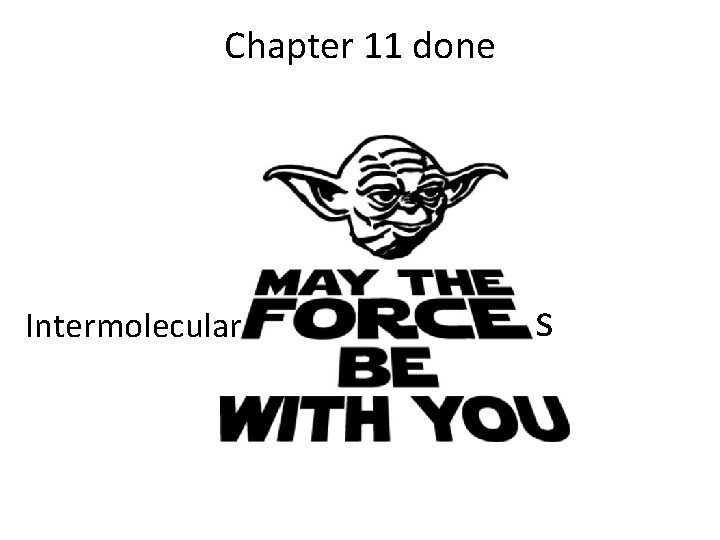Chapter 11 done Intermolecular s